# 【Unity3D开发小游戏】VVVVV游戏

@[TOC]

## 二、源码

UI资源和源代码请搜索QQ群：1040082875下载

## 三、正文

==Unity5.0.0f4==

### 1.摄像机设置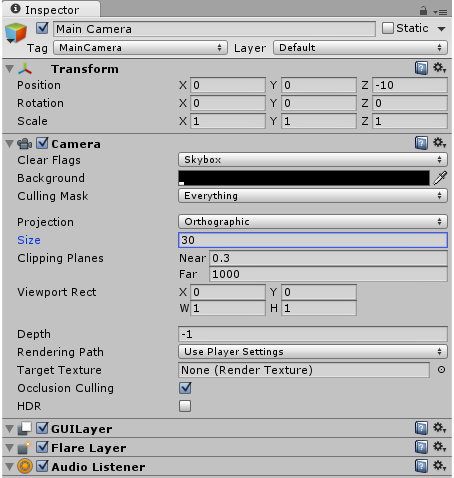### 2.平面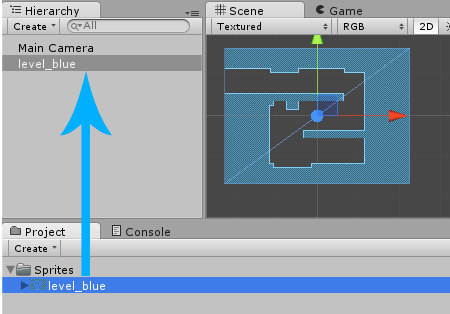### 3.平面物理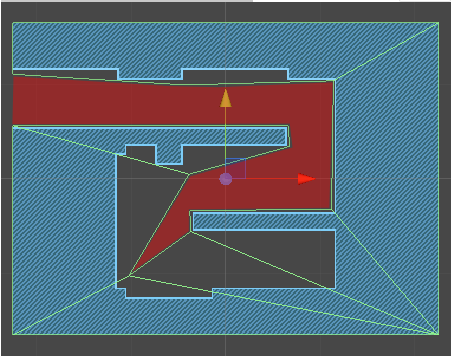Unity允许我们通过单击以下按钮修改多边形碰撞器：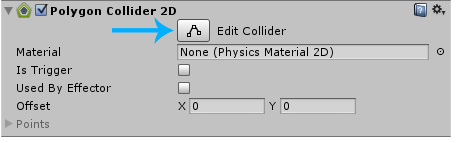之后，我们可以修改场景就像这样：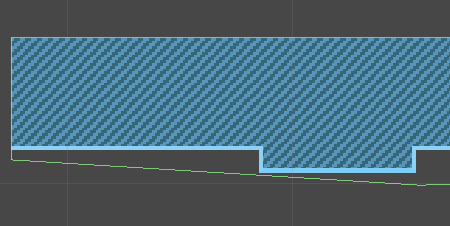让我们修改整个多边形，直到它完全符合我们的要求：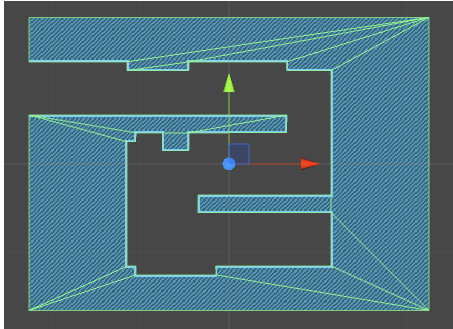现在平台的碰撞器是非常好的。

### 6.玩家动画

• 闲置右(切片0和1)
• 闲置左(切片2和3)
• 向右走(切片4和5)
• 向左走(切片6和7)

• 切片3-4命名为idle_left.anim
• 切片4-5命名为walk_right.anim
• 切片7-8命名为walk_left.anim

### 7.玩家动画状态机

• 闲置右
• 闲置左
• 向右走
• 向左走 我们还将添加Transitions，这样Unity就知道什么时候该从一种动画状态切换到另一种动画状态。

``````GetComponent<Animator>().SetInteger("DirX", 0);
``````

• idle_left到walk_left如果DirX小于0
• walk_left到idle_left如果DirX=0
• idle_left到walk_right如果DirX大于0
• walk_left到walk_right如果DirX大于0
• idle_right到walk_left如果DirX小于0
• walk_right到walk_left如果DirX小于0

### 9.玩家移动

``````using UnityEngine;
using System.Collections;

public class Player : MonoBehaviour {

// Update is called once per frame
void Update () {

}
}
``````

``````using UnityEngine;
using System.Collections;

public class Player : MonoBehaviour {
// Movement Speed
public float speed = 30;

// Update is called once per frame
void Update () {

}
}
``````

``````// Update is called once per frame
void Update () {
// Horizontal Movement
float h = Input.GetAxisRaw("Horizontal");
}
``````

``````// Update is called once per frame
void Update () {
// Horizontal Movement
float h = Input.GetAxisRaw("Horizontal");
GetComponent<Rigidbody2D>().velocity = Vector2.right * h * speed;
}
``````

``````// Update is called once per frame
void Update () {
// Horizontal Movement
float h = Input.GetAxisRaw("Horizontal");
GetComponent<Rigidbody2D>().velocity = Vector2.right * h * speed;

// Animation
GetComponent<Animator>().SetInteger("DirX", (int)h);
}
``````

``````// Update is called once per frame
void Update () {
// Horizontal Movement
float h = Input.GetAxisRaw("Horizontal");
GetComponent<Rigidbody2D>().velocity = Vector2.right * h * speed;

// Gravity Changes
if (Input.GetKeyDown(KeyCode.Space)) {
GetComponent<Rigidbody2D>().gravityScale *= -1;
transform.Rotate(0, 180, 180);
}

// Animation
GetComponent<Animator>().SetInteger("DirX", (int)h);
}
``````

``````public bool isGrounded() {
// Get Bounds and Cast Range (extents.y+20%)
Bounds bounds = GetComponent<Collider2D>().bounds;
float range = bounds.extents.y * 1.2f;

// Calculate a position slightly 'below' the collider
// (via transform.up so it's gravity tolerant)
Vector2 v = bounds.center - transform.up * range;

// Linecast upwards
RaycastHit2D hit = Physics2D.Linecast(v, bounds.center);

// Was there something in-between, or did we hit ourself?
return (hit.collider.gameObject != gameObject);
}
``````

``````// Gravity Changes
if (Input.GetKeyDown(KeyCode.Space) && isGrounded()) {
GetComponent<Rigidbody2D>().gravityScale *= -1;
transform.Rotate(0, 180, 180);
}
``````

### 10.检查点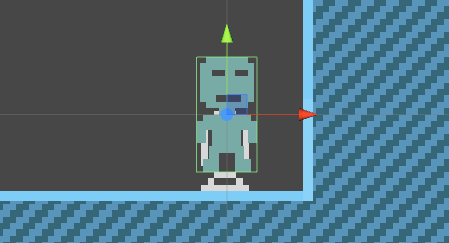``````public class Player : MonoBehaviour {
// Movement Speed
public float speed = 30;

// Last Checkpoint
Transform check;

...
}
``````

``````void OnTriggerEnter2D(Collider2D co) {
// Checkpoint?
if (co.name == "checkpoint")
check = co.transform;
}
``````

### 11.穿过V字尖峰

``````void OnCollisionEnter2D(Collision2D co) {
// Collided with a V?
if (co.collider.name == "V") {
// Reset Rotation, Gravity, Velocity and go to last Checkpoint
transform.rotation = Quaternion.identity;
GetComponent<Rigidbody2D>().gravityScale = Mathf.Abs(GetComponent<Rigidbody2D>().gravityScale);
GetComponent<Rigidbody2D>().velocity = Vector2.zero;
transform.position = check.position;
}
}
``````

• 更高层
• 背景音乐
• 故事
• 菜单
• 移动平台